# Math in Focus Grade 3 Cumulative Review Chapters 3 to 5 Answer Key

Go through the Math in Focus Grade 3 Workbook Answer Key Cumulative Review Chapters 3 to 5 to finish your assignments.

## Math in Focus Grade 3 Cumulative Review Chapters 3 to 5 Answer Key

Concepts and Skills

Add. (Lessons 3. 7, 3.2, and 3.3)

Question 1.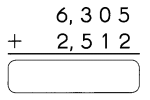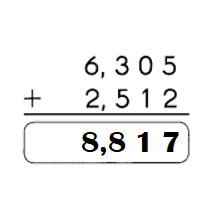Explanation:
6305 + 2512 = 8817.
(5 + 2 = 7 – Ones place
0 + 1 = 1 – tens place
3 + 5 = 8 – hundreds place
6+ 2 = 8- thousand place)

Question 2.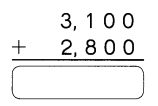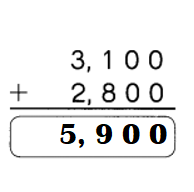Explanation:
3100 + 2800 = 5900.
(0 + 0 = 0 – Ones place
0 + 0 = 0 – tens place
1 + 8 = 9 – hundreds place
3+ 2 = 5 – thousand place)

Subtract. (Lessons 4. 1, 4.2 and 4.3)
Question 3.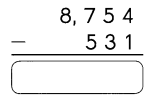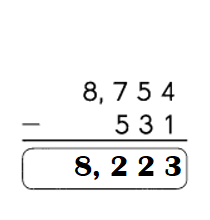Explanation:
7754 – 531 = 8223.
(4 – 1 = 3 – Ones place
5 – 3 = 2 – tens place
7 – 5 = 2 – hundreds place
8 – 0 = 8 – thousand place)

Question 4.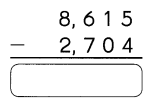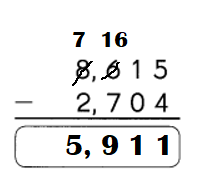Explanation:
8615 – 2704 = 5911.
(5 – 4 = 1 – Ones place
1 – 0 = 1 – tens place
6 – 7 =(borrowed from thousands place) 16 – 7 = 9 – hundreds place
8-1 = 7 – 2 = 5 – thousand place)

Fill in the missing numbers.
Question 5.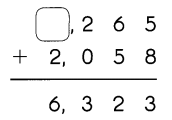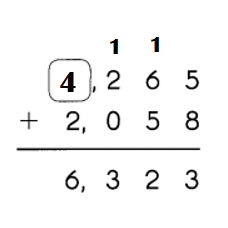Explanation:
Missing number = 2 + ?? = 6
=> ?? = 6 – 2
=> ?? = 4.

Question 6.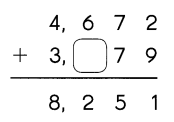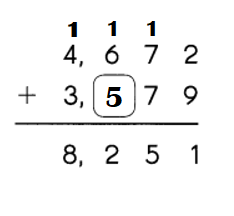Explanation:
Missing number =1 +  6 + ?? = 12
=> 7 + ?? = 12
=> ?? = 12 – 7
=> ?? = 5.

Question 7.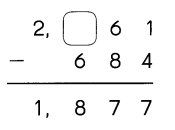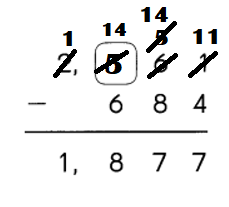Explanation:
Missing number =
(?? + 1)(tens place number borrowed 1 from hundreds place number) – 6 = 8
=> (?? + 1) = 6 + 8
=> ?? + 7 = 8
=> ?? = 8 + 7
=> ?? = 15.

Question 8.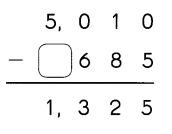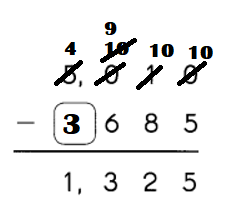Explanation:
Missing number =
(5 – 1) (hundreds place number borrowed from thousands place number) – ?? = 1.
=> (5 – 1) – ?? = 1
=> 4 – 1 = ??
=> 3 = ??.

Complete. (Lesson 4.4)
Question 9.
Gabriel and Sue are at an amusement park.
a. Gabriel throws two darts at a target board. The difference between the two numbers is 75. Circle the two numbers.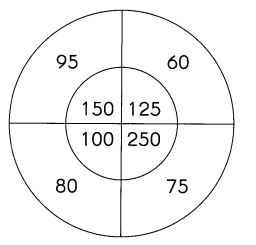The two numbers whose difference  is 75 are 150 and 75.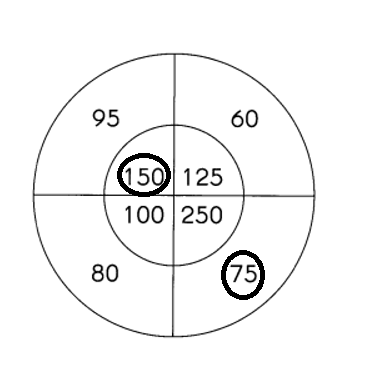Explanation:
Differences:
1. 150 – 95 = 55.     150 – 60 = 90.    150 – 75 = 75.    150 – 80 = 70.
2. 125 – 95 = 30.     125 – 60 = 65.    125 – 75 = 50.   125 – 80 = 45.
3. 250 – 95 = 175.    250 – 60 = 190.    250 – 75 = 175.   250 – 80 = 80.
4. 100 – 95 =  5.      100 – 60 = 40.      100 – 75 = 25.      100 – 80 = 20.

b. Sue throws two darts at another target board. The difference between the two numbers is 2,700. Circle the two numbers.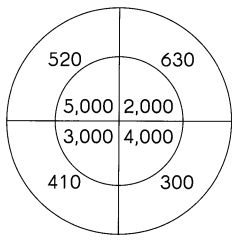The two numbers whose difference  is 75 are 3000 and 300.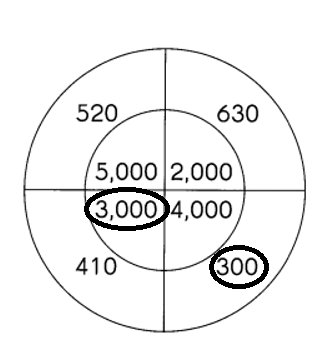Explanation:
Differences:
1. 5000 – 520 = 4480.     5000 – 630 = 4370.    5000 – 300 = 4700.    5000 – 410 = 4590.
2. 2000 – 520 = 1480.     2000 – 630 = 1370.    2000- 300 = 1700.   2000 – 410 = 1590.
3. 4000 – 520 = 3480.    4000 – 630 = 3370.    4000 – 300 = 3700.   4000 – 410 = 3590.
4. 3000 – 520 =  2480.      3000 – 630 = 2370.      3000 – 300 = 2700.      3000 – 410 = 2590.

Problem Solving
Question 10.
5,476 people ride Bus D in March.
1,496 fewer people ride Bus E in the same month.
a. How many people ride Bus E in March?
Number of people ride Bus E in the same month = 3980.

Explanation:
Number of people ride Bus D in March = 5476.
Number of fewer people ride Bus E in the same month = 1496.
=> Number of people ride Bus E in the same month = Number of people ride Bus D in March – Number of fewer people ride Bus E in the same month
=> 5476 – 1496
=> 3980.

b. How many people ride both buses in March?
Total number of people ride both buses in March = 9456.

Explanation:
Number of people ride Bus D in March = 5476.
Number of people ride Bus E in the same month = 3980.
Total number of people ride both buses in March = Number of people ride Bus D in March + Number of people ride Bus E in the same month
= 5476 + 3980
= 9456.

Question 11.
A supermarket has 1,213 apples. 368 are green apples.
a. How many of the apples are red?
Number of red apples = 845.

Explanation:
Total number of apples super market has = 1213.
Number of green apples = 368.
Number of red apples = Total number of apples super market has – Number of green apples
= 1213 – 368
= 845.

b. How many more red apples than green apples does the supermarket have?
477 more red apples than green apples the supermarket has.

Explanation:
Number of red apples = 845.
Number of green apples = 368.
Difference:
Number of red apples – Number of green apples
= 845 – 368
= 477.

Question 12.
2,500 people visit the Children’s Museum on Monday. On Tuesday, there were 532 more people at the museum than on Monday. What is the total number of visitors for both days?
Total number of people visit the Children’s Museum on both days = 5532.

Explanation:
Number of people visit the Children’s Museum on Monday = 2500.
On Tuesday, there were 532 more people at the museum than on Monday.
=>Number of people visit the Children’s Museum on Tuesday = Number of people visit the Children’s Museum on Monday + 532
=> 2500 + 532
=> 3032.
Total number of people visit the Children’s Museum on both days = Number of people visit the Children’s Museum on Monday + Number of people visit the Children’s Museum on Tuesday
= 2500 + 3032
= 5532.

Question 13.
Michael has 754 songs in his audio device. He has 98 more songs than Peter. How many songs do they have in all?